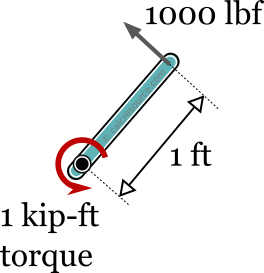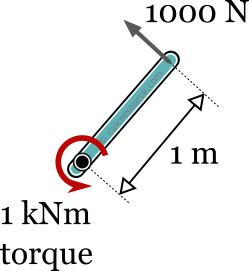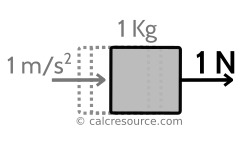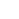## kNm - kip ft converter

Enter the moment or torque in either of the next two fields and get it converted instantly, from kilonewton-meters to kilopound-feet (kNm to kip-ft), or reversely, from kilopound-feet to kilonewton-meters (kip-ft to kNm).### Conversion Formulas

1 kip-ft ≈ 1.35582 kNm

1 kNm ≈ 0.737562 kip-ft

#### Precise conversion:

1 kip-ft = 1.3558179483314004 kNm

1 KNm ≈ ¾ kip-ft

## Definitions

### The kilopound-feet [kip-ft]

The kilopound-feet (symbolized kip-ft or kip.ft or kip ft) is a unit of moment or torque, according to the Imperial and US customary system of units. It is the product of kilopound [kip] which is one thousand pounds [lbf], a unit of force, with feet [ft], which is a unit of length. The kip-ft is equivalent to the torque applied from 1000 pound force [lfb] exercised at a 1 foot distance from the rotation axis.A torque of 1 kip-ft is equivalent to a 1000lbf force exercised at 1ft distance from rotation axis

Pound force [lbf] is defined as the force of gravity on the earth's surface upon a mass equal to one avoirdupois pound. Its equivalence to the respective metric unit, the Newton [N] is: 1 lbf = 4.4482216152605 N. The above definition depends on the earth's gravitational acceleration g, which varies from place to place, but is conventionally averaged to g ≈ 32.1740 ft/s2, at sea level.A force of 1 lbf corresponds to the weight of one pound mass at sea level

Foot [ft] is a unit of length for the Imperial and US customary system of units. It was historically conceived as the length of a human male foot. Today, however, it is conventionally defined through the meter SI unit as:

1ft = 0.3048m

The conversion rule between kilopound-feet and kilonewton-meters (kip-ft to kNm) can be derived, based on the conversion rules between the two components of the unit: the force and the length. It is:

1 lbf = 4.4482216152605 N

Therefore, 1 kip, which is 1000 pound becomes also:

1 kip = 4.4482216152605 KN

As a result, 1kip x 1ft would be:

1kip x 1ft = 4.4482216152605KN x 0.3048m

Finally:

1kip-ft = 1.3558179483314004 kNm

This is the conventionally equivalent of 1 kip-ft in kNm.

#### Kilopound-feet and other units of moment/torque

The relationship between kilopound-feet [kip-ft] and other units of moment are shown in the following table (i.e. how many kip-ft makes every unit in the table):

A quick example how to use the above table is given here. Assuming, you need to find the relationship between Newton-meter [Nm] and kilopound-feet [kip-ft], the following equation is obtained from the table:

1 Nm = 7.37562 10-4 kip-ft

or

1 Nm = 0.000737562 kip-ft

### The kilonewton-meter [kNm]

The kilonewton-meter (symbolized kNm or KNm) is a unit of moment or torque, according to the SI (Systeme International) system of units. It is the product of kilonewton [kN] which is one thousand Newtons [N], a unit of force, with meter [m], which is a unit of length. The kNm is equivalent to the torque applied from 1000 Newton force exercised at a 1 meter distance from the rotation axis.A torque of 1 kNm is equivalent to a 1000l Newton force exercised at 1m distance from rotation axis

Newton [N] is a unit of force according to the SI system of units. It is a derived unit, based on the kilogram [Kg], a unit of mass, the metre [m], a unit of length and the second [s], a unit of time. It is defined as the force required to accelerate a mass equal to 1 Kg by 1 m/s2, according to the Newton's second law of motion:

where the force, the mass and the acceleration.A force of 1 Newton accelerates with 1m/s2 a mass of 1kg

Using the Newton's second law of motion with earth's gravitational acceleration g (for this purpose approximated to g ≈ 9.806 m/s2), we can relate the Newton force unit with the weight of a unit mass (equal to 1 kg). We obtain:

1 kg x 9.807 m/s2 ≈ 9.807 N

or

1 N ≈ 0.102 kg m/s2

This means, that a force of 1 N, is equivalent to the weight of a mass equal to 102 gr, at earth's sea level. Similarly, 10 N are equivalent to the weight of 1.02 kg mass. These are rules of thumb, useful for many practical purposes. In reality however, earth's gravity depends on the location (altitude and geographical latitude), and cannot be considered an invariant constant.

Meter or metre [m] is the base unit of length for the SI system of units. It was originally conceived as 1/10,000,000 of the distance along earth's meridian from the equator to the North Pole (a full longitude circle of earth is approximately equal to 40,000 km). Today, it is defined as the distance traveled by light in vacuum in seconds. This definition offers the advantage of invariance through space and time but on the other hand is impractical for everyday use.

#### Kilonewton-meters and other units of moment/torque

The relationship between kilonewton-meters [kNm] and other units of force is shown in the following table (i.e. how many kNm makes each one of the units in the table):

Some quick instructions for using the above table are given here. Assuming, you need to find the relationship between kilopound inch [kip-in] and kilonewton-meters [kNm], the following equation is obtained from the table:

1 kip-in = 0.112985 KNm

### How to convert kilopound-feet to kilonewton-meters (kip-ft to kNm)

• Multiply moment in kip-ft with 1.35582
• The result is the force in kNm

For example to convert 80 kip-ft to kNm we have: 80x1.35582 ≈ 108.47 kNmFor a precise conversion multiply kip-ft with 1.3558179483314004 to get kNm

### How to convert kilonewton-meters to kilopound-feet (kNm to kip ft)

• Multiply moment in kNm with 0.737562
• The result is the force in kip-ft

For example to convert 400 kNm to kip-ft we have: 400x0.737562 ≈ 295.02 kip-ftFor a more precise conversion multiply kNm with 0.7375621492772653639 to get kip-ft

### Conversion table from kilonewton-meters to kilopound-feet (KNm to kip-ft)

In the following table some typical moment/torque values in kNm are converted to kip-ft. These conversions are approximated with 4 significant digits. More precise values can be obtained from the calculator in the top of the page.

### Conversion table from kilopound-feet to kilonewton-meters (kip-ft to kNm)

In the following table some typical moment/torque values in kip-ft are converted to kNm. These conversions are approximated with 4 significant digits. More precise values can be obtained from the calculator in the top of the page.